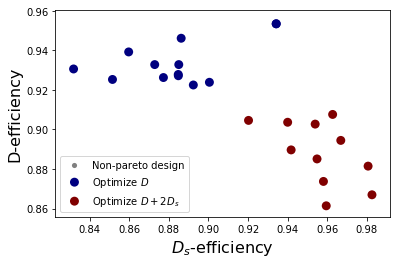# Generate D-efficient designs

This notebook contains example code from the article Two-level designs to estimate all main effects and two-factor interactions by Eendebak, P. T. and Schoen, E. D. This example shows how to generate D-efficient designs with a user-specified optimization function.

:

import numpy as np
import oapackage
import oapackage.Doptim

%matplotlib inline


Define the class of designs to generate.

:

run_size = 40
number_of_factors = 7
factor_levels = 2
strength = 0
arrayclass = oapackage.arraydata_t(factor_levels, run_size, strength, number_of_factors)
print("We generate D-efficient designs with %d rows and %d columns\n" % (run_size, number_of_factors))

We generate D-efficient designs with 40 rows and 7 columns



Generate a single D-efficient design using $$\alpha=(1,2,0)$$ as the parameters for the optimization function. For details on this parameter and its corresponding optimization function, see Two-Level Designs to Estimate All Main Effects and Two-Factor Interactions.

:

alpha = [1, 2, 0]
scores, design_efficiencies, designs, ngenerated = oapackage.Doptim.Doptimize(
arrayclass, nrestarts=30, optimfunc=alpha, selectpareto=True
)

Doptim: optimization class 40.2-2-2-2-2-2-2
Doptimize: iteration 0/30
Doptimize: iteration 29/30
Doptim: done (11 arrays, 0.4 [s])

:

print("\nGenerated %d designs, the efficiencies for these designs are:" % len(designs))
for ii, d in enumerate(designs):
dd = d.Defficiencies()
print("array %d: D-efficiency %.4f, Ds-efficiency %.4f" % (ii, dd, dd))

D = [d.Defficiency() for d in designs]
best = np.argmax(D)
print("\nThe design with the highest D-efficiency (%.4f) is:\n" % D[best])

designs[best].transposed().showarraycompact()


Generated 11 designs, the efficiencies for these designs are:
array 0: D-efficiency 0.8815, Ds-efficiency 0.9807
array 1: D-efficiency 0.9076, Ds-efficiency 0.9628
array 2: D-efficiency 0.8670, Ds-efficiency 0.9827
array 3: D-efficiency 0.8945, Ds-efficiency 0.9669
array 4: D-efficiency 0.9027, Ds-efficiency 0.9540
array 5: D-efficiency 0.8851, Ds-efficiency 0.9549
array 6: D-efficiency 0.8737, Ds-efficiency 0.9581
array 7: D-efficiency 0.9036, Ds-efficiency 0.9400
array 8: D-efficiency 0.8614, Ds-efficiency 0.9595
array 9: D-efficiency 0.8897, Ds-efficiency 0.9418
array 10: D-efficiency 0.9046, Ds-efficiency 0.9203

The design with the highest D-efficiency (0.9076) is:

0010011001011001010101011101101100011101
0011010111100101111100010111001100100010
1011011111001010100010111001000010011010
0101100001100111110011010001001100111110
0011100010001101001011101101110100010001
0011110000011011101001110011101010101000
1000110011101000101110001001101111001101


Optimizing with a different optimization target leads to different D-efficient designs. Below we compare the sets of designs generated with optimization target [1,0,0] and [1,2,0].

:

scores0, design_efficiencies0, designs0, _ = oapackage.Doptim.Doptimize(
arrayclass, nrestarts=30, optimfunc=[1, 0, 0], selectpareto=True
)

Doptim: optimization class 40.2-2-2-2-2-2-2
Doptimize: iteration 0/30
Doptimize: iteration 29/30
Doptim: done (13 arrays, 0.4 [s])

:

def combineEfficiencyData(lst):
data = np.zeros((0, 4))

for jj, dds in enumerate(lst):
dds_index = np.hstack((dds, jj * np.ones((len(dds), 1))))
data = np.vstack((data, dds_index))
return data

design_efficiencies_combined = combineEfficiencyData([design_efficiencies0, design_efficiencies])
plot_handles = oapackage.generateDscatter(
design_efficiencies_combined, ndata=3, lbls=["Optimize $D$", "Optimize $D+2D_s$"], verbose=0
)

Pareto: 23 optimal values, 24 objects<Figure size 432x288 with 0 Axes>

[ ]: